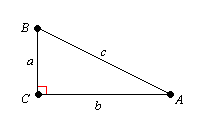# Trigonometric Ratios

"Trigon" is Greek for triangle , and "metric" is Greek for measurement. The trigonometric ratios are special measurements of a right triangle (a triangle with one angle measuring $90°$ ). Remember that the two sides of a right triangle which form the right angle are called the legs , and the third side (opposite the right angle) is called the hypotenuse .

There are three basic trigonometric ratios: sine , cosine , and tangent . Given a right triangle, you can find the sine (or cosine, or tangent) of either of the non- $90°$ angles.

Example:

Write expressions for the sine, cosine, and tangent of $\angle A$ .The length of the leg opposite $\angle A$ is $a$ . The length of the leg adjacent to $\angle A$ is $b$ , and the length of the hypotenuse is $c$ .

The sine of the angle is given by the ratio "opposite over hypotenuse." So,

$\mathrm{sin}\angle A=\frac{a}{c}$

The cosine is given by the ratio "adjacent over hypotenuse."

$\mathrm{cos}\angle A=\frac{b}{c}$

The tangent is given by the ratio "opposite over adjacent."

$\mathrm{tan}\angle A=\frac{a}{b}$

Generations of students have used the mnemonic " SOHCAHTOA " to remember which ratio is which. ( S ine: O pposite over H ypotenuse, C osine: A djacent over H ypotenuse, T angent: O pposite over A djacent.)

## Other Trigonometric Ratios

The other common trigonometric ratios are:

Example:

Write expressions for the secant, cosecant, and cotangent of $\angle A$ .The length of the leg opposite $\angle A$ is $a$ . The length of the leg adjacent to $\angle A$ is $b$ , and the length of the hypotenuse is $c$ .

The secant of the angle is given by the ratio "hypotenuse over adjacent". So,

$\mathrm{sec}\angle A=\frac{c}{b}$

The cosecant is given by the ratio "hypotenuse over opposite".

$\mathrm{csc}\angle A=\frac{c}{a}$

The cotangent is given by the ratio "adjacent over opposite".

$\mathrm{cot}\angle A=\frac{b}{a}$